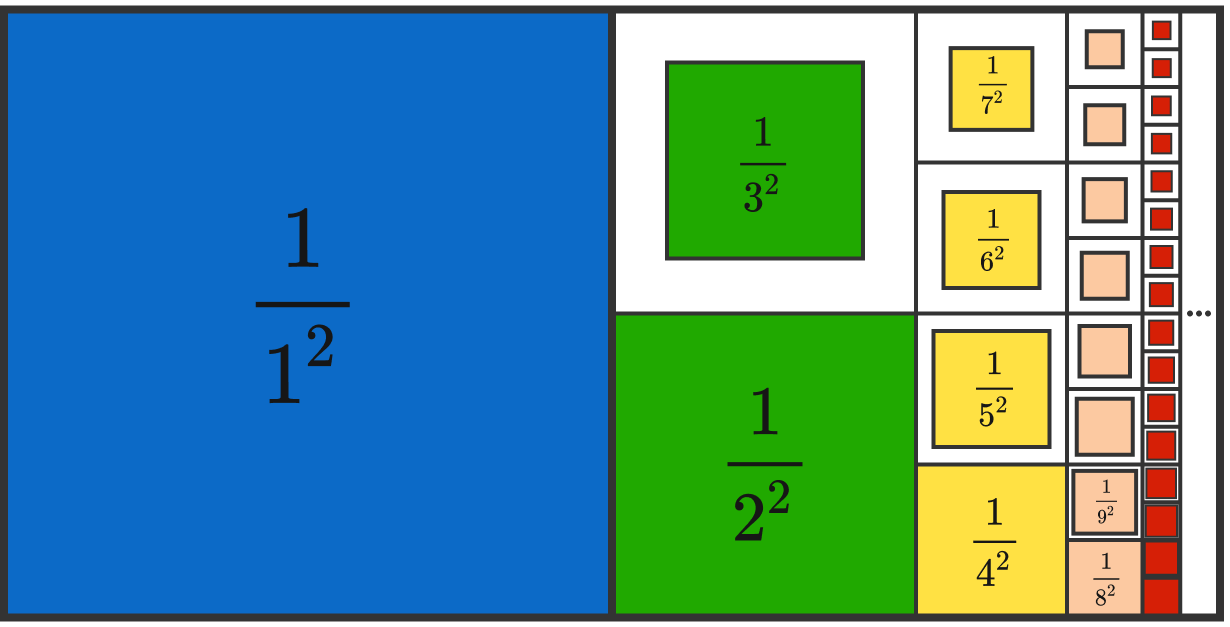# Reciprocal Sums

Calculus Level 1

It is known that $\frac{ 1}{1} + \frac{1}{2} + \frac{1}{3} + \cdots$ is infinite.

What about

$\frac{1}{1^2} + \frac{1}{2^2} + \frac{1}{3^2} + \cdots \ ?$

Hint:×

Problem Loading...

Note Loading...

Set Loading...